# Basic Arithmetic : Median

## Example Questions

← Previous 1 3

### Example Question #1 : Median

Find the median of the following numbers: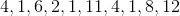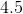Explanation:

If you reorganize the numbers and put them in ascending order, you get: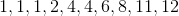.

The median is the number which falls in the middle of a set. Our set hasentries therefore to find the true middle of the set we will need to take entry five and six and find its mean. We do this by adding entry five and entry six together and then dividing by two: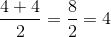Therefore the median of our set is.

### Example Question #21 : Basic Statistics

Consider the following number set: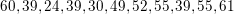What is the mean of the median and mode of the above set?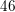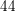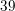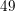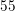Explanation:

To solve this problem, we need to remember the definitions of mean, median, and mode:

Mean is the average of all numbers of a given set: we find this by adding all the numbers together and dividing by the total number of numbers there are.

Median is the middle of a data set: if we align all the numbers of a set, and cross off the numbers on each end until we reach the middle, that middle number is the median of the data set.

Mode is the number which appears most commonly in a data set.

Therefore, to find the median and mode of the above set, if would be helpful to arrange the set in numerical order: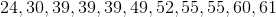The median is the number in the direct middle of the set. In this case, that would be. The mode is the number which appears most often in the set. Since it appears three times,is the mode of our set. Our final step is to find the mean of these two numbers: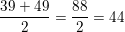Our final answer is therefore.

### Example Question #21 : Basic Statistics

Tom has not been doing very well in his algebra class. Recently, he has received test scores of,,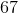,,, and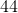What is the median of Tom's test scores?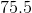Explanation:

The correct answer to this question is 50. In order to approach the problem, you must first start by placing the numbers in order from least to greatest: 27, 34, 44, 56, 67, and 84.

Since there are six numbers in the set, there is not a single number in the middle of the set to be the median.

In this case, we have two numbers in the middle of the set: 44 and 56.

In order to obtain the median, we must take the average of these two numbers.

We do this by completing the following equation: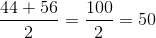### Example Question #21 : Basic Statistics

Joanna had 6 history tests this semester. Her scores on the tests were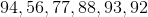.

What was her median score?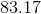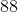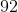Explanation:

To find the median, first put all the numbers in numerical order.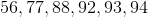The median is our middle number. Because this set has 6 numbers, our median will fall in between 88 and 92. Average those two numbers to find the median.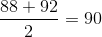### Example Question #21 : Basic Statistics

What is the median for the following set of numbers?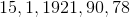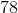Explanation:

The median is the middle number in a set of numbers listed in ascending order.

First, list the given numbers from smallest to largest.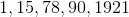Now, the middle number here isbecause it has 2 terms on either side.

### Example Question #1 : How To Find Median

Determine the median, from the set of numbers: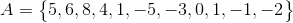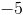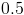Explanation:First put your set in numerical order, from smallest to largest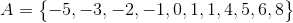Median refers to the number in the middle, so if you count in from both sides the middle number of the set is### Example Question #1 : How To Find Median

Find the median of the following set of numbers: 3, 5, 18, 6, 3.Explanation:

The median of a set of numbers is the number that falls in the middle when the numbers are arranged from smallest to largest: 3, 3, 5, 6, 18.  The number that falls exactly in the middle of this set is 5, which is the median.

### Example Question #1 : Median

We are given the following number set:

8, 6, 10, 15, 7, 15 ,5, 14, 9, 5, 19, 18, 9, 16, 9

Find the median.Explanation:

The median is the middle number of an ordered number set. By ordered number set, I mean that the numbers are arranged from lowest to largest. In this problem, we can arrange the number set from lowest to largest so that it is rewritten as

5, 5, 6, 7, 8, 9, 9, 9, 10, 14, 15, 15, 16, 18, 19

It looks like the middle-most number is 9.  Therefore, 9 is the median.

### Example Question #1 : Median

Find the median of the set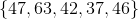.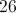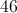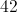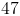Explanation:

The median of a set of numbers is simply the middle number in the ordered set. To find it, we can first put the set in order from least to greatest (greatest to least works just as well). The set can now be read as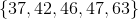Now, it is clear that the median number is 46. Don't confuse median and mean! The mean, or average value, is the result of the sum of all the values divided by the number of terms in the set.

### Example Question #12 : How To Find Median

What is the median of the following numbers?

12,15,93,32,108,22,16,21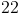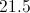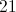Explanation:

To find the median, first you arrange the numbers in order from least to greatest.

Then you count how many numbers you have and divide that number by two. In this case 12,15,16,21,22,32,93,108= 8 numbers.

So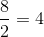Then starting from the least side of the numbers count 4 numbers till you reach the median number ofThen starting from the greatest side count 4 numbers until you reach the other median number ofFinally find the mean of the two numbers by adding them together and dividing them by two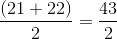to find the median number of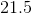.

← Previous 1 3

### All Basic Arithmetic Resources